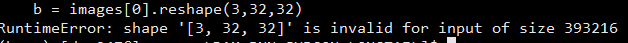# Image Display in Pytorch

Hi,
Any suggestion to display an image having a dimension of (128, 3, 32, 32) in Pytorch,
It generates the following error. I think batch size (128) should be removed but how?

Invalid shape (128, 3, 32, 32) for image data

have you tried `image[n]` n = 1 or … to 128

I m using
b = images
plt.imshow(b)

Now problem is the shape of image= 128,3,32,32
So how to discard 128 (bsz).

what is the shape of image? usually its batch =128, rgb =3, width=32, height =32. Not sure what your 5th dimension is (shape of image) could you post the error log and shape of image (image.shape in case of numpy).
also try image

Shape of image is 128,3,32,32

The following code snippet could help to understand:

def train(train_loader, model, criterion, optimizer, epoch ):

``````model.train()

losses = AverageMeter()

rows = 2
cols = 2
fig=plt.figure()
axes = []
for idx, (images, labels) in enumerate(train_loader):
data_time.update(time.time() - end)

print('.........Size of image before unsqueeze : ',images.shape)
b = images.reshape(3,32,32)
npimg = b.numpy()

plt.imshow(npimg)
plt.show()
exit()
``````

The error is:The `image` should be a single item, not a batch of items. If the shape of that is `[128,3,32,32]` then that would be the shape of your data. If you print out `image.shape` you will get the shape of the data in the batch. If you would like to change the shape of your data you should probably look at the construction of the `__getitem__` in your dataset and see what the shape of the data that is being put in there.

If `image` shape is `(128, 3, 32, 32)` then you have another dimension and each batch has the shape `(batch_dim, 128, 3, 32, 32)` i.e. every batch has n `128x3x32x32`-dimensional Tensors.

Are you sure this is what you want?

If so, you can plot `image[0, i, ...]` for `i = 0, 1, ..., 127`

And remember that if you want to plot a 3-channel image with matplotlib you need it in shape `(x, y, channels)` e.g.

``````# First image of the first batch
img_plot = image[0, 0, ...].permute(1, 2, 0)
plt.imshow(img_plot)
``````
1 Like